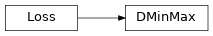# DMinMax¶

Inheritance Diagramclass ashpy.keras.losses.DMinMax(from_logits=True, label_smoothing=0.0)[source]

Bases: tensorflow.python.keras.losses.Loss

Implementation of MinMax Discriminator loss as tf.keras.losses.Loss.

$L_{D} = - \frac{1}{2} E [\log(D(x)) + \log (1 - D(G(z))]$

Methods

 __init__([from_logits, label_smoothing]) Initialize the loss. call(d_real, d_fake) Compute the MinMax Loss.

Attributes

 reduction Return the reduction type of this loss.
__init__(from_logits=True, label_smoothing=0.0)[source]

Initialize the loss.

Return type: None
call(d_real, d_fake)[source]

Compute the MinMax Loss.

Play the DiscriminatorMinMax game between the discriminator computed in real and the discriminator compute with fake inputs.

Parameters: d_real (tf.Tensor) – Real data. d_fake (tf.Tensor) – Fake (generated) data. Tensor tf.Tensor – Output Tensor.
reduction

Return the reduction type of this loss.

Return type: ReductionV2 :py:classes:tf.keras.losses.Reduction – Reduction.